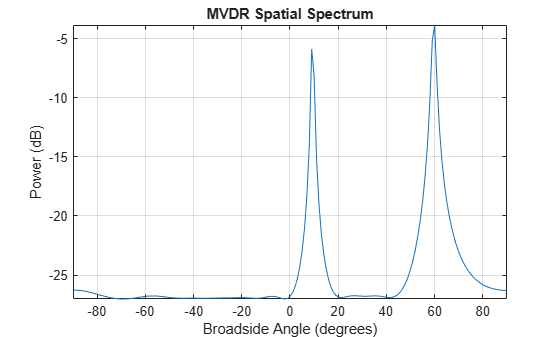# plotSpectrum

System object: phased.MVDREstimator
Package: phased

Plot spatial spectrum

## Syntax

```plotSpectrum(estimator) plotSpectrum(estimator,Name,Value) hl = plotSpectrum(___) ```

## Description

`plotSpectrum(estimator)` plots the spatial spectrum resulting from the most recent execution of the object.

`plotSpectrum(estimator,Name,Value)` plots the spatial spectrum with additional options specified by one or more `Name,Value` pair arguments.

`hl = plotSpectrum(___)` returns the line handle in the figure.

## Input Arguments

 `H` Spatial spectrum estimator object.

### Name-Value Arguments

Specify optional pairs of arguments as `Name1=Value1,...,NameN=ValueN`, where `Name` is the argument name and `Value` is the corresponding value. Name-value arguments must appear after other arguments, but the order of the pairs does not matter.

Before R2021a, use commas to separate each name and value, and enclose `Name` in quotes.

 `NormalizeResponse` Set this value to `true` to plot the normalized spectrum. Setting this value to `false` plots the spectrum without normalization. Default: `false` `Title` Character vector to use as figure title. Default: `''` `Unit` Plot units, specified as `'db'`, `'mag'`, or `'pow'`. Default: `'db'`

## Examples

expand all

First, estimate the DOAs of two signals received by a standard 10-element ULA with element spacing of 1 meter. The antenna operating frequency is 150 MHz. The actual direction of the first signal is 10° in azimuth and 20° in elevation. The direction of the second signal is 60° in azimuth and −5° in elevation. Then, plot the MVDR spatial spectrum.

Create the signals with added noise. Then, create the ULA System object™.

```fs = 8000; t = (0:1/fs:1).'; x1 = cos(2*pi*t*300); x2 = cos(2*pi*t*400); array = phased.ULA('NumElements',10,'ElementSpacing',1); array.Element.FrequencyRange = [100e6 300e6]; fc = 150.0e6; x = collectPlaneWave(array,[x1 x2],[10 20;60 -5]',fc); noise = 0.1*(randn(size(x)) + 1i*randn(size(x)));```

Construct MVDR estimator System object.

```estimator = phased.MVDREstimator('SensorArray',array,... 'OperatingFrequency',fc,'DOAOutputPort',true,'NumSignals',2);```

Estimate the DOAs.

```[y,doas] = estimator(x + noise); doas = broadside2az(sort(doas),[20 -5])```
```doas = 1×2 9.5829 60.3813 ```

Plot the spectrum.

`plotSpectrum(estimator)`